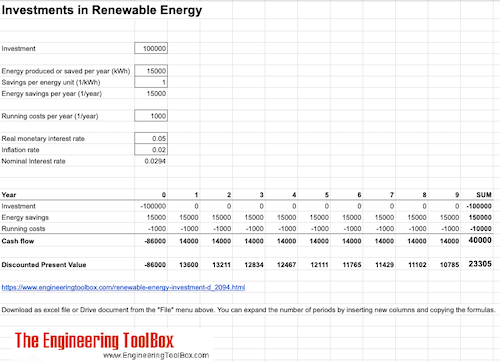Engineering ToolBox - Resources, Tools and Basic Information for Engineering and Design of Technical Applications!

# Investments in Renewable Energy

## Calculate investments in renewable energy.

Renewable energy - like the sun or wind - are available for free, but the equipment required to use the energy is not.

The economy in a renewable energy project can be calculated with alternative methods - the most common are

• payback time
• simple annual method
• discounted cash flow

### Payback Time

Payback time - the time it takes to save or earn an amount equal to the investment - can be calculated as

t = I / A

= I / (E c)                 (1)

where

t = payback time (years)

I = investment

A = annual net income - or savings - from the investment

E = energy produced or saved per year (kWh/year)

c = cost or savings per energy unit (1/kWh)

#### Example - An Investment in a Windmill Generator

An amount of 100000 is invested in a windmill generator. The windmill is assumed to produce 8000 kWh/year. The cost to buy this energy from the local grid is 1 per kWh

The savings on produced energy can be calculated as

A = (8000 kWh/year) (1 1/kWh)

= 8000

The payback time can be calculated to

t = 100000 / 8000

= 12.5 year

#### Renewable Energy - Payback Time Calculator

I - investment

E - produced or saved energy per year (kWh/year)

c - energy cost per kWh (kWh-1)

### Simple Annual Method

The economy alternatively be evaluated by calculating the cost of the energy produced or saved. The cost of energy can be calculated as

c = (I / y + C) / E                    (2)

where

c = cost or savings per energy unit (1/kWh)

I = investment

C = average annual running costs (1/year)

E = energy produced or saved per year (kWh/year)

#### Example - An Investment in Solar Panels

An amount of 50000 is invested in a solar panel system with estimated annual energy production of 4000 kWh/year. The lifespan for the system is estimated to 25 year. The average annual cost to operate the system is assumed to be 1200 per year.

The cost of the produced energy can be calculated as

c = ((50000) / (25 years) + (1200 1/year)) / (4000 kWh/year)

= 0.8 1/kWh

#### Renewable Energy - Simple Annual Method Calculator

I - investment

C - average annual running costs ()

E - energy produced or saved per year (kWh/year)

### Discounted Cash Flow

Since money earned or spend in the future is not worth the same as money earned or spend today - it is common to discount the future cash flow back to a present value. The present value of the cash flows in a renewable project can be calculated as

P = F0 / (1 + i)0 + F1 / (1 + i)1 + F2 / (1 + i)2 + .... + Fn / (1 + i)n                    (3)

where

P = present value

F = cash flow

i = real interest rate

A renewable energy project typically start with a huge investment in the beginning continuing with smaller income and running costs. The cash flow for a typical year can be expressed as

Fn = En cn - Cn                    (3a)

where

Fn = cash flow per year (1/year)

En = energy produced or saved per year (kWh/year)

cn = costs or savings per energy unit (1/kWh)

Cn = running costs (1/year)

The real interest can be calculated as

in = (1 + im n)/ (1 + ii n) - 1                      (3b)

where

in = nominal interest rate

im n = real monetary interest rate

ii n = rate of inflation

#### Example - Investment in Renewable Energy and Discounted Cash Flow

An investment of 100000 saves 15000 kWh/year in energy. The energy cost is 1 per kWh, running costs to operate the system is 1000, inflation rate is 0.02 (2%) and the monetary interest rate is 0.05 (5%).

A discounted present value for the project over 10 years can be calculated to 23305 as indicated in the Google spreadsheet below.## Related Topics

• Economics - Engineering economics - cash flow diagrams, present value, discount rates, internal rates of return - IRR, income taxes, inflation.

## Engineering ToolBox - SketchUp Extension - Online 3D modeling!

Add standard and customized parametric components - like flange beams, lumbers, piping, stairs and more - to your Sketchup model with the Engineering ToolBox - SketchUp Extension - enabled for use with the amazing, fun and free SketchUp Make and SketchUp Pro .Add the Engineering ToolBox extension to your SketchUp from the SketchUp Pro Sketchup Extension Warehouse!

Translate

## Privacy

We don't collect information from our users. Only emails and answers are saved in our archive. Cookies are only used in the browser to improve user experience.

Some of our calculators and applications let you save application data to your local computer. These applications will - due to browser restrictions - send data between your browser and our server. We don't save this data.

## Citation

• Engineering ToolBox, (2018). Investments in Renewable Energy. [online] Available at: https://www.engineeringtoolbox.com/renewable-energy-investment-d_2094.html [Accessed Day Mo. Year].

Modify access date.

. .

#### Scientific Online Calculator3 10